# AP Statistics : How to find correlation

## Example Questions

### Example Question #1 : How To Find Correlation

Which of the following correlation coefficients indicates the strongest relationship between variables?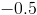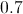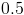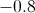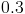Explanation:

Correlation coefficients range from 1 to -1.  The closer to either extreme, the stronger the relationship.  The closer to 0, the weaker the relationship.

### Example Question #2 : How To Find Correlation

It is found that there is a correlation of exactly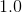between two variables. Which of the following is incorrect?

Correlation is measured on a scale of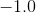toAll of the answer choices are correct

There is enough evidence, with a correlation of, to assert that one variable causes the other

There is a strong association between the two variables.

The association between the two variables is positive

There is enough evidence, with a correlation of, to assert that one variable causes the other

Explanation:

Under no circumstance will correlation ever equate to causation, regardless of how strong the correlation between two variables is. In this case, all other answer choices are correct.

### Example Question #3 : How To Find Correlation

In a medical school, it is found that there is a correlation of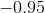between the amount of coffee consumed by students and the number of hours students sleep each night. Which of the following is true?

i. There is a positive association between the two variables.

ii. There is a strong correlation between the two variables.

iii. Coffee consumption in medical school students causes students to sleep less each night.

i and ii

i and iii

iii only

ii only

i, ii, and iii

ii only

Explanation:

Since the correlation is negative, there must be a negative association between the two variables (therefore statement i is incorrect). Statement ii is correct since a correlation of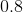toon an absolute value scale oftois considered to be a strong correlation. Statement iii is incorrect since correlation does not mean causation.

### Example Question #4 : How To Find Correlation

Which of the following shows the least correlation between two variables?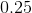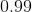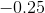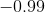Explanation:

The strength of correlation is measured on an absolute value scale oftowithbeing the least correlated andbeing the most correlated. The positive or negative in front of the correlation integer simply determines whether or not there is a positive or negative correlation between the variables.

A correlation ofmeans that there is no correlation at all between two variables.

### Example Question #5 : How To Find Correlation

Which of the following correlation coefficients implies the strongest relationship between variables: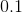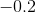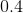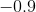Explanation:

A high correlation coefficient regardless of sign implies a stronger relationship. In this casehas a stronger negative relationship than the positive relationship described by a value of### Example Question #6 : How To Find Correlation

A national study on cell phone use found the following correlations:

-The correlation between the number of texts sent each day and a person's average credit card debt is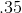.

-The correlation between the number of texts sent each day and the number of books read each month is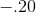.

Which of the following statements are true?

i. As the number of texts sent each day increases, average credit card debt increases.

ii. Sending more texts causes people to read less.

iii. A person's average credit card debt is related more strongly to the number of texts sent each day than the number of books read each month is related to the number of texts sent each day.

ii

ii and iii

i and ii

iii

i and iii

iii is correct because the absolute values of the correlations indicate which correlation is stronger.is a stronger correlation than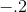.